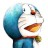# 杰拉斯的博客

## [整理]ACM模拟题详解（3）——数论（续）杰拉斯 | 时间：2012-02-17, Fri | 4,284 views

## 5、Prime Ring Problem

Problem Description

A ring is compose of n circles as shown in diagram. Put natural number 1, 2, ..., n into each circle separately, and the sum of numbers in two adjacent circles should be a prime.

Note: the number of first circle should always be 1.

Input

n (0 < n < 20).

Output

The output format is shown as sample below. Each row represents a series of circle numbers in the ring beginning from 1 clockwisely and anticlockwisely. The order of numbers must satisfy the above requirements. Print solutions in lexicographical order.

You are to write a program that completes above process.

Print a blank line after each case.

Sample Input

6

8

Sample Output

Case 1:

1 4 3 2 5 6

1 6 5 2 3 4

Case 2:

1 2 3 8 5 6 7 4

1 2 5 8 3 4 7 6

1 4 7 6 5 8 3 2

1 6 7 4 3 8 5 2

1+2

1+4

1+6

1+2+3

1+2+5

1+4+3

1+6+5

##```/*
* Prime Ring Problem
*/
public static void test4(int n){
int values[] = new int[n];
// 初始化
for(int i=0;i<n;i++){
values[i] = i+1;
}

// 表示遍历过程中可能的解
List list = new ArrayList();

// 处理后面的n-1个数字，1永远是第一个
for(int i=1;i<n;i++){
List temp = list;
list = new ArrayList();
// 对于每个可能的解，得到下一层节点
for(int j=0;j<temp.size();j++){
int tempValues[]=(int[])temp.get(j);
// 考虑所有可能的组合
for(int k=i;k<n;k++){
if(isPrime(tempValues[i-1]+tempValues[k])){
// 创建新的状态，并复制原来的值
int[] newValues = Arrays.copyOf(tempValues, tempValues.length);
// 交换i和k处的值
if(i!=k){
int change = newValues[i];
newValues[i] = newValues[k];
newValues[k] = change;
}
// 把新状态添加到列表中
}
}
}
}
// 输出结果
for(int i=0;i<list.size();i++){
int[] tempValues = (int[])list.get(i);
if(isPrime(tempValues+tempValues[n-1])){
for(int j=0;j<n;j++){
System.out.print(tempValues[j]+" ");
}
System.out.println();
}
}
System.out.println(list.size());
}
```

```/*
* Prime Ring Problem（深度优先）
*/
public static void test5(int n){
// 数组的前n个元素表示环中的数字，第n+1个数据表示数组中前n+1个元素是满足条件的
int values[] = new int[n+1];
// 初始化
for(int i=0;i<n;i++){
values[i] = i+1;
}
values[n]=1;

// 表示遍历过程中可能的解
List list = new ArrayList();
StringBuffer sb = new StringBuffer();
while(list.size()>0){
// 取出第一个元素
int tempValues[]=(int[])list.get(0);
// 表示处理到第几层，第一层用0表示
int index=tempValues[n];
// 遍历并生成所有可能的下一层节点
for(int k=tempValues[n];k<n;k++){
if(isPrime(tempValues[index-1]+tempValues[k])){
// 如果是最后一层并且，最后一个数和第一个数的和
// 也是质数，则输出结果
if(index==n-1 && isPrime(tempValues[index]+1)){
for(int j=0;j<n;j++){
sb.append(tempValues[j]+" ");
}
sb.append("/n");
// 分批输出
if(sb.length()>10000){
System.out.print(sb.toString());
sb=new StringBuffer();
}
continue;
}
// 创建新的状态，并复制原来的值
int[] newValues = Arrays.copyOf(tempValues, tempValues.length);
// 交换i和k处的值
if(index!=k){
int change = newValues[index];
newValues[index] = newValues[k];
newValues[k] = change;
}
newValues[n] = newValues[n]+1;
// 把新状态添加到列表中,放在最前面，深度优先，
// 如果采用广度优先，则应该放到最后面
}
}
// 从list中删除当前的节点
list.remove(tempValues);
}
System.out.print(sb.toString());
}
```

## 6、人见人爱A^B

Problem Description

Input

Output

Sample Input

2 3

12 6

6789 10000

0 0

Sample Output

8

984

1

```/*
* A的B次方的后3位
*/
public static int test6(int a,int b){
// 保留后3位
int temp = a%1000;
int result = temp;
for(int i=1;i<b;i++){
result = (result*temp)%1000;
}
return result;
}
```